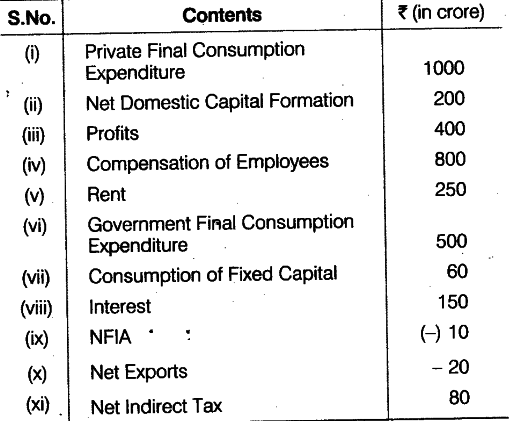# Calculate Gross National Product

Calculate Gross National Product at Factor Cost from the following data by:
(a) Income method
(b) Expenditure method(a) By income method,
\$GN{{P}_{FC}}\$ = Compensation of Employees + (Profits + Rent + Interest)

• Consumption of Fixed Capital + Net Factor Income from Abroad = 800+ (400+250+ 150)+60+ (- 10)
= Rs. 1650 crore
(b) By expenditure method,
\$GN{{P}_{FC}}\$ = Private Final Consumption Expenditure+Government Final Consumption Expenditure + Net Domestic Capital Formation + Consumption of Fixed Capital + Net Export - Net Indirect Taxes + Net Factor Income from Abroad
= 1000 + 500 + 200 + 60 + (- 20) - 80 + (-10) =Rs (1760 - 110) crore =Rs. 1650 crore